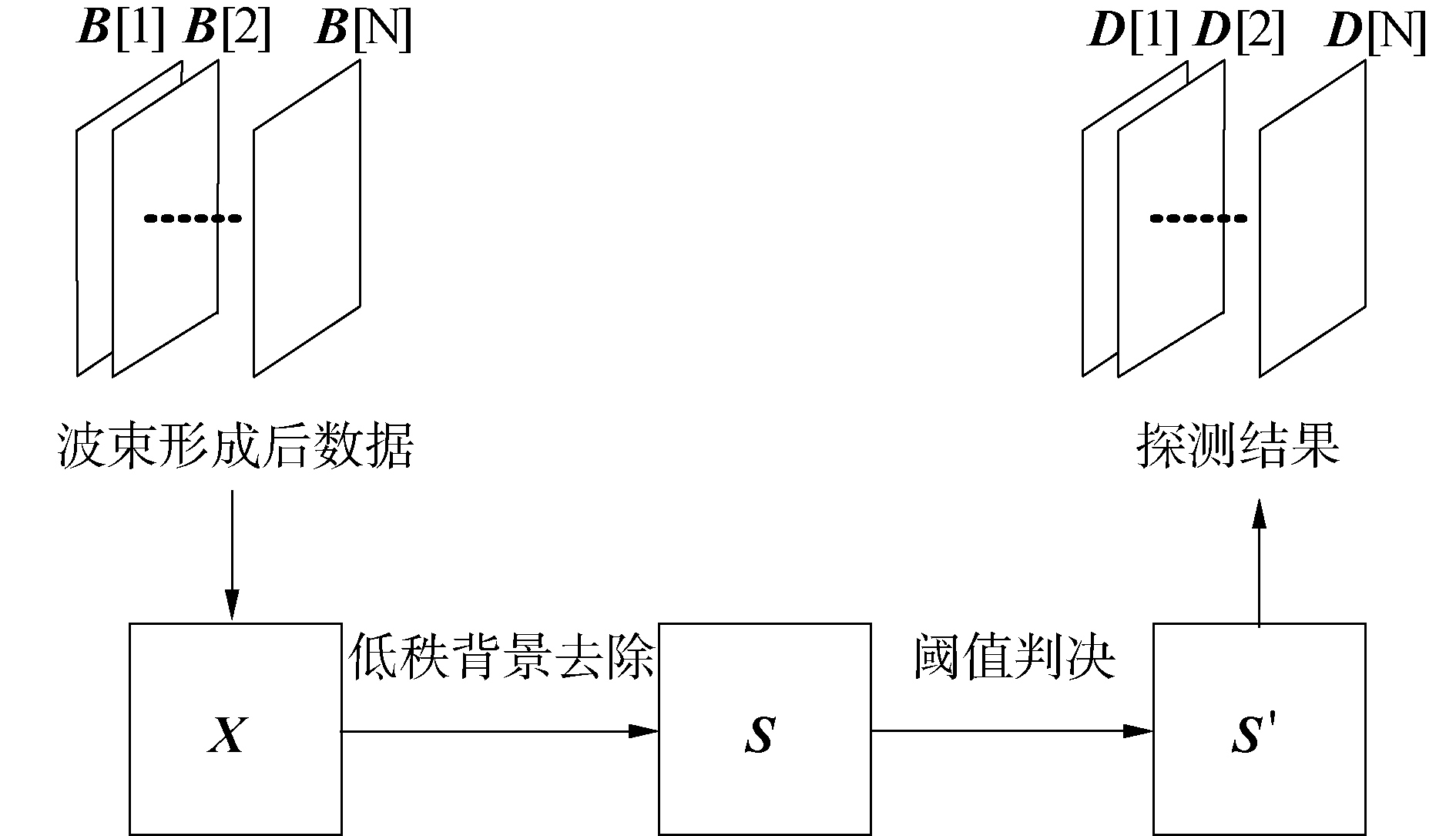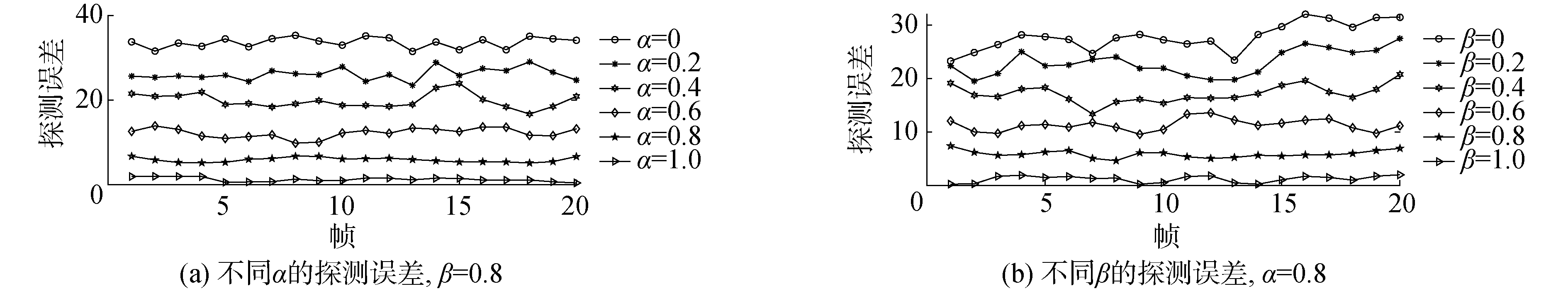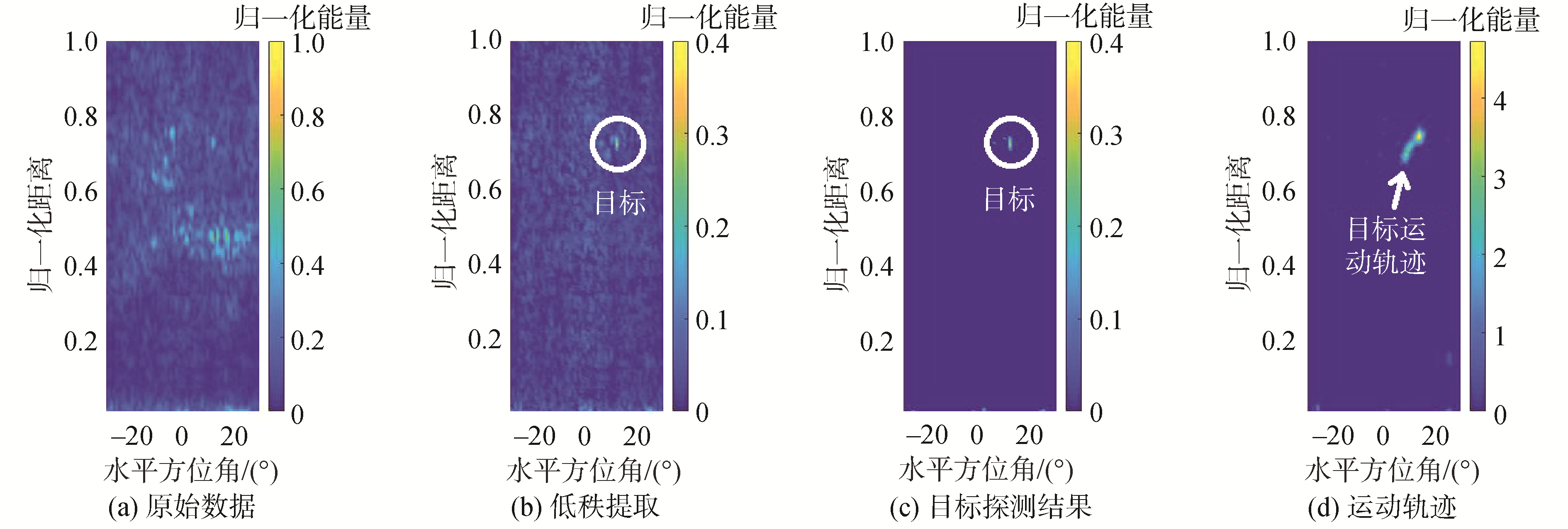﻿ 基于随机算法的抗混响目标探测方法
«上一篇文章快速检索 高级检索

 哈尔滨工程大学学报2020, Vol. 41Issue (2): 277-281  DOI: 10.11990/jheu.2019050390

### 引用本文LIU Bing, YIN Jingwei, ZHU Guangping, et al. A target detection method in reverberation environment based on stochastic algorithm[J]. Journal of Harbin Engineering University, 2020, 41(2): 277-281. DOI: 10.11990/jheu.201905039.### 文章历史

1. 哈尔滨工程的大学 水声技术重点实验室, 黑龙江 哈尔滨 150001;
2. 海洋信息获取与安全工业和信息化部重点实验室(哈尔滨工程大学), 黑龙江 哈尔滨 150001;
3. 哈尔滨工程大学 水声工程学院, 黑龙江 哈尔滨 150001

A target detection method in reverberation environment based on stochastic algorithm
LIU Bing 1,2,3, YIN Jingwei 1,2,3, ZHU Guangping 1,2,3, GUO Longxiang 1,2,3
1. Acoustic Science and Technology Laboratory, Harbin Engineering University, Harbin 150001, China;
2. Key Laboratory of Marine Information Acquisition and Security(Harbin Engineering University), Ministry of Industry and Information Technology, Harbin 150001, China;
3. College of Underwater Acoustic Engineering, Harbin Engineering University, Harbin 150001, China
Abstract: In order to solve the problem of detecting a moving target under strong reverberation, a new detection method was proposed in this paper. This method takes advantage of the correlation of multi-frame data. Stochastic algorithm is used to carry out linear measurement of multi-frame data in the method. The low-rank structure of multi-frame data is extracted by bilateral projection to suppress reverberation, thus moving target detection is realized. Results of numerical simulations and an experiment showed that the proposed method can accurately detect a moving target under strong reverberation. Compared with traditional methods, this method has an obvious advantage and is very suitable for detection in serious reverberation environment such as harbor waters.
Keywords: moving target    active detection    target detection    reverberation    stochastic algorithm    low-rank    low-rank structure    low-rank matrix

1 抗混响目标探测方法 1.1 低秩结构提取

 $\left\{\begin{array}{l} \boldsymbol{Y}=\mathit{\pmb{\Omega X}} \\ \boldsymbol{\tilde{Y}}=\mathit{\pmb{\tilde{\Omega}}} \boldsymbol{X}^{\mathrm{T}} \end{array}\right.$

 $\boldsymbol{L}=\tilde{\boldsymbol{Y}}^{\mathrm{T}}\left(\mathit{\pmb{\Omega}} \tilde{\boldsymbol{Y}}^{\mathrm{T}}\right)^{\dagger} \boldsymbol{Y}$ (1)

 ${\mathit{\boldsymbol{Y}}_1} = {\mathit{\pmb{\varOmega} \pmb{X}}}$ (2)

 ${\mathit{\boldsymbol{Y}}_2} = {\mathit{\boldsymbol{Y}}_1}{\mathit{\boldsymbol{X}}^{\rm{T}}} = \mathit{\boldsymbol{ \boldsymbol{\varOmega} X}}{\mathit{\boldsymbol{X}}^{\rm{T}}}$ (3)

 ${\mathit{\boldsymbol{Y}}_3} = {\mathit{\boldsymbol{Y}}_2}\mathit{\boldsymbol{X}} = \mathit{\mathit{\pmb{\varOmega} \boldsymbol{X}}}{\mathit{\boldsymbol{X}}^{\rm{T}}}\mathit{\boldsymbol{X}}$ (4)

 $\boldsymbol{L}=\boldsymbol{Y}_{2}^{\mathrm{T}}\left(\boldsymbol{Y}_{2} \boldsymbol{Y}_{2}^{\mathrm{T}}\right)^{\dagger} \boldsymbol{Y}_{3}$ (5)
1.2 探测方法

 $\boldsymbol{x}_{n}=\left[\boldsymbol{b}_{1}[n] ; \boldsymbol{b}_{2}[n] ; \cdots ; \boldsymbol{b}_{j}[n] ; \cdots ; \boldsymbol{b}_{N_{\theta}}[n]\right]$

 $\boldsymbol{X}=\left[\boldsymbol{x}_{1}, \boldsymbol{x}_{2}, \cdots, \boldsymbol{x}_{n}, \cdots, \boldsymbol{x}_{N}\right]$

 $\boldsymbol{X}=\boldsymbol{L}+\boldsymbol{S}$ (6)

 \begin{aligned} \boldsymbol{S^{\prime}} &=\operatorname{Th}_{\xi}[\boldsymbol{S}] \\ \boldsymbol{Z} &=\boldsymbol{S}-\boldsymbol{S^{\prime}} \end{aligned}

 $S_{i, j}^{\prime}=\operatorname{Th}_{\xi}\left[S_{i, j}\right]=\left\{\begin{array}{ll} S_{i, j}, & S_{i, j}>\xi \\ 0, &其他 \end{array}\right.$

 $\mathit{\boldsymbol{X}}{\rm{ = }}\mathit{\boldsymbol{L}}{\rm{ + }}\mathit{\boldsymbol{S}}{\rm{' + }}\mathit{\boldsymbol{Z}}$Download: 图 1 数据处理流程 Fig. 1 Processing flow of the beamforming data

1) 设置输入参数。数据序列{B[n]}，最大迭代次数K，门限ξ，相对误差上限ε

2) 初始化。令S=0，生成感知矩阵Ω，向量化{B[n]}→X, X=XT

3) 迭代。

for k =1: K // K次迭代

$\mathit{\boldsymbol{\tilde X}}$=XS//更新矩阵

Y1=Ω$\mathit{\boldsymbol{\tilde X}}$, Y2=Y1$\mathit{\boldsymbol{\tilde X}}$T, Y3=Y2$\mathit{\boldsymbol{\tilde X}}$//双边投影

L=Y2T(Y2Y2T)Y3 //低秩结构提取

S=XL //计算数据矩阵稀疏部分

S=Th0[S]//非负约束

If ‖XSLF/‖XF < ε, break; end

//判断是否终止迭代，‖·‖ F为Frobenius范数

end

4) 阈值检测。S′=Thξ[S]；

5) 逆向量化并输出探测结果。S′=S′T, S′→{D[n]}。

2 数值仿真与实验

 $\mathit{\boldsymbol{R}}[n] = {\rm{ }}\beta \mathit{\boldsymbol{R}}\left[ 0 \right] + (1 - {\rm{ }}\beta )\mathit{\boldsymbol{A}}[n]$ (7)

 ${A_{i, j}}[n] = {\rm{ }}\alpha {A_{i, j}}[n{\rm{ }} - 1] + (1 - {\rm{ }}\alpha )v$ (8)

 $T_{i, j}[n]=\left\{\begin{array}{l} 0.5 \;\;目标出现位置\\ 0\;\;其他位置 \end{array}\right.$Download: 图 2 抗混响目标探测仿真结果 Fig. 2 Simulation results of target detection with reverberation suppression

 ${\rm{E}}[n] = {\left\| {\mathit{\boldsymbol{T}}[n] - \mathit{\boldsymbol{D}}[n]} \right\|_{\rm{F}}}$Download: 图 3 抗混响目标探测探测误差 Fig. 3 Detection error of target detection with reverberation suppressionDownload: 图 4 抗混响目标探测实验结果 Fig. 4 Experiment results of target detection with reverberation suppression

3 结论

1) 随机算法作为一种高效的算法，能够对矩阵的低秩部分进行的提取。

2) 本文对多帧接收数据组成的矩阵进行了低秩结构提取，并在处理过程中加入了非负约束条件，最终以迭代的方式成功实现了混响和目标的分离。本文提出的方法在理论上是可行的。

3) 本文所提出的方法在不同条件下，性能有所差异。数值仿真表明，各帧混响的相关性越强，目标探测效果就越好。

4) 本文所提出的方法在实际中也具有良好的探测性能，具有良好的推广前景。

  杨益新, 韩一娜, 赵瑞琴, 等. 海洋声学目标探测技术研究现状和发展趋势[J]. 水下无人系统学报, 2018, 26(5): 369-387. YANG Yixin, HAN Yi'na, ZHAO Ruiqin, et al. Ocean acoustic target detection technologies:a review[J]. Journal of unmanned undersea systems, 2018, 26(5): 369-387. (0)  张杨梅.水下小目标主动探测关键技术研究[D].西安: 西北工业大学, 2017. ZHANG Yangmei. Research on the key technologies of active detection of underwater small target[D]. Xi'an: Northwestern Polytechnical University, 2017. http://cdmd.cnki.com.cn/Article/CDMD-10699-1018792669.htm (0)  李晖宙, 刘正红, 毛盾. 基于蛙人探测声纳序列图像的水下小目标跟踪算法[J]. 舰船电子工程, 2018, 38(2): 26-30, 34. LI Huizhou, LIU Zhenghong, MAO Dun. Underwater small target tracking algorithm based on diver detection sonar image sequences[J]. Ship electronic engineering, 2018, 38(2): 26-30, 34. DOI:10.3969/j.issn.1672-9730.2018.02.007 (0)  KAY S, SALISBURY J. Improved active sonar detection using autoregressive prewhiteners[J]. The journal of the acoustical society of America, 1990, 87(4): 1603-1611. DOI:10.1121/1.399408 (0)  TRUCCO A, DI SERIO S, MURINO V. Buried object detection by auto-regressive pre-whitening[C]//Proceedings of MTS/IEEE Riding the Crest into the 21st Century. Seattle, WA, USA, 1999: 126-132. (0)  BESBES H, JEBARA S B. The pre-whitened NLMS: a promising algorithm for acoustic echo cancellation[C]//Proceedings of the 9th International Conference on Electronics, Circuits and Systems. Dubrovnik, Croatia, 2002: 875-878. (0)  BESBES H, JEBARA S B. A variable step size pre-whitened sign algorithm with quantized normalizing factor. Application to acoustic echo cancellation[C]//Proceedings of the 2004 12th European Signal Processing Conference. Vienna, Austria, 2004: 709-712. (0)  SHUI Penglang, LIU Hongwei, BAO Zheng. Range-spread target detection based on cross time-frequency distribution features of two adjacent received signals[J]. IEEE transactions on signal processing, 2009, 57(10): 3733-3745. DOI:10.1109/TSP.2009.2029715 (0)  XIA Yunlong, PIAO Shengchun, FU Yongqing. Combination of WVD with TRM on detection of LFM signal in inhomogeneous medium[C]//Proceedings of the 2009 4th IEEE Conference on Industrial Electronics and Applications. Xi'an, China, 2009: 3864-3867. (0)  YU Ge, PIAO Shengchun, HAN Xiao. Fractional Fourier transform-based detection and delay time estimation of moving target in strong reverberation environment[J]. IET radar, sonar & navigation, 2017, 11(9): 1367-1372. (0)  WOOLFE F, LIBERTY E, ROKHLIN V, et al. A fast randomized algorithm for the approximation of matrices[J]. Applied and computational harmonic analysis, 2008, 25(3): 335-366. DOI:10.1016/j.acha.2007.12.002 (0)  FAZEL M, CANDES E, RECHT B, et al. Compressed sensing and robust recovery of low rank matrices[C]//Proceedings of the 2008 42nd Asilomar Conference on Signals, Systems and Computers. Pacific Grove, CA, USA, 2008: 1043-1047. (0)  ZHOU Tianyi, TAO Dacheng. GoDec: Randomized low-rank & sparse matrix decomposition in noisy case[C]//Proceedings of the 28th International Conference on Machine Learning. Bellevue, WA, USA, 2011. (0)